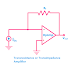In the field of analog electronics, you may hear many times the terms 'Transresistance' and 'Transconductance'. In fact, when we study about electronic amplifier we must have knowledge about them. So in this article, we are going to learn about them as much as possible in a simple language.

First of all, let's know what is conductance and resistance. Conductance is the property of an object or material by virtue of which it allows the flow of current through it. More conductance means more current flow. On the other hand, Resistance is the property of an object or material by virtue of which it resists or blocks the current flow through it. More resistance means less current flow.

Resistance is just the opposite of conductance, so conductance is reciprocal of resistance. Resistance and Conductance are may be the internal property of any object or material or may be created by various factors in an electrical system.

## Transresistance

The term 'Transresistance' comes from Transfer Resistance. It is also known as Mutual Resistance. While relating to the transconductance, it is the dual of transconductance.

Transresistance refers to the ratio between changes in the voltage at two output points and changes in the current through two input points.

Rm = ΔVout / ΔIIn

Here, the Rm is the symbol of Transresistance. The R denotes the resistance and subscript m denotes the mutual. ΔVout is the change in output voltage and ΔIIn is the change in input current.

The transresistance is measured in Ohm which is the SI unit of it. And it is denoted by the symbol Ω.

Actually, the term transresistance is used for the DC power system when it comes to the AC power system it is called Transimpedance( also known as transfer impedance).

Uses with Example: 1. Transresistance is measured in some amplifiers where the input current controlled the output voltage. These amplifiers are also known as Transresistance Amplifiers or Transimpedance Amplifiers. A common example of it is when an operational amplifier is used by connecting its output to the inverting input with a feedback path.
2. Transresistance is also measured for the Current Controlled Voltage source(CCVS).

## Transconductance

The term 'Transconductance' comes from Transfer Conductance. It is also known as Mutual Conductance. While relating to Transresistance, it is half of Transresistance.

Transconductance refers to the ratio between changes in the current through two output points and changes in the voltage at two input points.

Gm = ΔIout / ΔVIn

Here, the Gm is the symbol of Transconductance. The G denotes the conductance and subscript m denotes the mutual. ΔIout is the change in output current and ΔVIn is the change in input voltage.

The SI unit of Transconductance is Siemens which is denoted by the symbol S.

The equivalent term of Transconductance used for the AC power system is Transadmittance or Transfer Admittance.

Uses with Example: 1. Transconductance is measured for some amplifiers where the input voltage control the output current. These amplifiers are also known as Transconductance Amplifiers or Gm Amplifiers. An amplifier using a Field Effect Transistor(FET) is an example of it where the Gate to Source Voltage controlled the Drain Current.
2. Transconductance is also measured for Voltage Controlled Current Source(VCCS).

Learn about Transresistance and TransconductanceReviewed by Author on May 09, 2023 Rating: 5